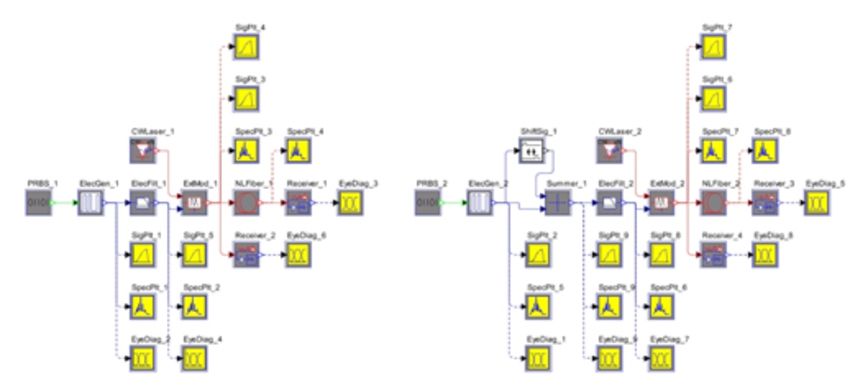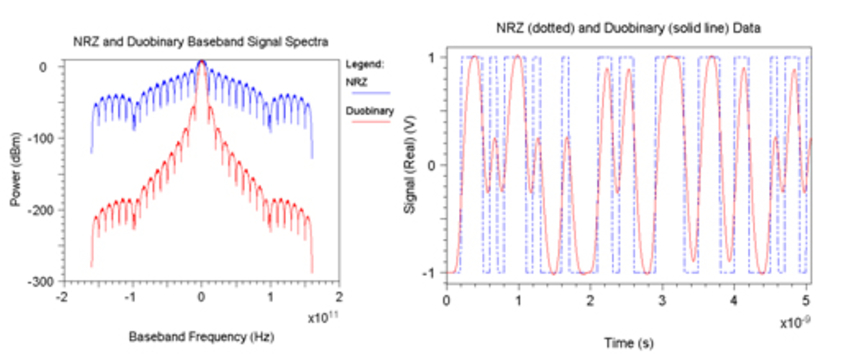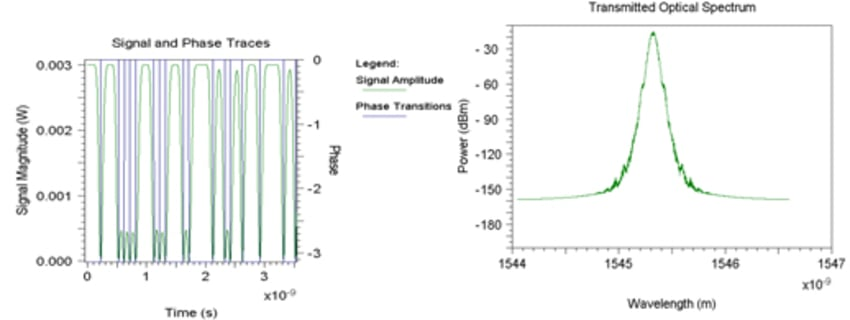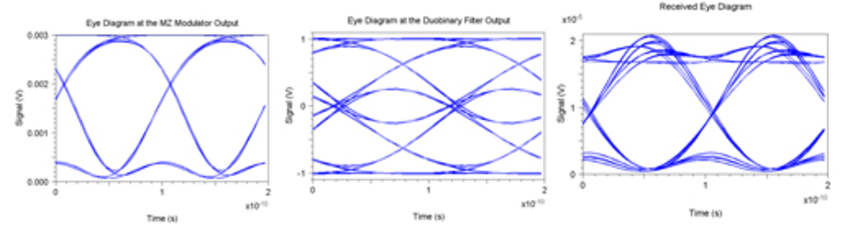# RSoft Application Gallery Note: Study of Duobinary Transmitter

## Tool Used: OptSim

In this example, we investigate generation and transmission of duobinary data. We simulate a single-channel (1550nm) 90-km long OC-192 link as shown below.It is known that a duo-binary filter can be approximated by a low-pass filter with half-power cut-off at approximately one- fourth the data rate. At this cut-off frequency, the spectral occupancy of the modulated optical field is restricted to [f0 ± (Bit rate)/2], where f0 is the nominal continuous wave (CW) laser frequency. The receiver's electrical bandwidth requirement is identical for both the conventional non-return to zero (NRZ) and the amplitude-modulated phase-shift keyed (AM-PSK) duo-binary system here. The frequency spectrum of an ideal duo-binary signal exhibits the first nulls at [f0 ± (Bit rate)/2]. We can band-limit the signal at [f0 ± (Bit rate)/2] while preserving at the same time, enough information for later reconstruction of the signal. The duo-binary filter used for this example is a fifth order low-pass Bessel filter. The topology is shown on the left in the figure above. The right hand side topology of the above figure is an alternative approach of generating duobinary signals where an ideal NRZ is passed through a delay-and-add circuit followed by an optional bandlimiting filter of bandwidth (Bit rate)/2.

The figure below shows signal plots (left) and base-band spectra plots (right) for binary NRZ and duo-binary NRZ transmissions respectively. The duo-binary filter compresses the spectral occupancy of the signal.The figure below shows transmitted optical spectrum of duobinary data at the output of MZ modulator (left) and also shown on the right is signal amplitude and 1800 phase transitions at the output of MZ modulator.Finally, the figure below shows single scan eye diagrams at following points of interest: (1) at the output of duobinary filter, (2) at the output of MZ modulator and (3) at the receive end of a 90-km standard single mode fiber (SMF) link with 17ps/nm.km dispersion.